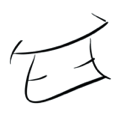# Gravity — Javascript Universe

Back in 2001, after an idle browse I wrote a basic javascript universe that animating images around the screen, with respect to their gravitational influences on each other. The code is now long lost but I was recently reminded of it while thinking of ideas for mobile phone games. So here’s my recreation.

We'll need some particles that have size, position & velocity. Velocity (which is speed in a given direction) will be represented with an x and y speed giving us the direction and overall speed.

`var particles = [ { size: { radius: 40, mass: 1000 }, position: { x: 390, y: 230 }, velocity: { x: 0, y: 0 }, fillStyle: '#F3B553' }, ...];`

Now we need to set up a recurring loop to keep track of all of the particles and to move them to their new positions. This loop will be responsible for;

• Updating the particles velocities dependent on their influences.
• Applying the particles velocities to their position (i.e. moving them).
• Re-drawing the canvas.

We can do this using the window.setInterval() method as follows;

`window.setInterval(universeLoop, 1000 / 15);`

Note: The interval here is set for 15 times a second regardless of your frame rate. In this example increasing or decreasing this will directly affect the speed at which the particles move. This can be fixed by introducing the concept of Game Time which I'll discuss later.

`var universeLoop = function () { var i, len = particles.length; for (i = 0; i < len; i++) { update(particles[i]); } clear(); for (i = 0; i < len; i++) { draw(particles[i]); }}`

For drawing we'll simply use the canvas’ arc method to render a circle or ellipse.

To update a particle we'll calculate any velocity changes using “Newton’s Law of Universal Gravitation”.
F = G x ((m1 x m2) / r2)

`var newtonsLawOfUniversalGravitation = function (mass1, mass2, distance) { var force = g * ((mass1 * mass2) / Math.pow(distance, 2)); var attraction2 = force / mass2; return attraction2;};`

In order to do so we'll need to know a particles mass and the distance between it and the influencing particles, which we can calculate using “Pythagoras theorem”.
a2 + b2 = c2

`var getDistance = function (x1, y1, x2, y2) { var a = Math.abs(x1 - x2); var b = Math.abs(y1 - y2); return Math.sqrt(Math.pow(a, 2) + Math.pow(b, 2));};`

This will give us an attraction in a specific direction, i.e. towards the influencing particle. Which we can split into its x and y components using trigonometry.

`var direction = Math.atan2(x1 - x2, y1 - y2);var attractionX = Math.sin(direction) * attraction;var attractionY = Math.cos(direction) * attraction;`

Then it’s a simple case of updating the particles velocity with the force of attraction.

--

--

--

## More from InfectiousGrin

http://infectiousgr.in

Love podcasts or audiobooks? Learn on the go with our new app.## InfectiousGrin

http://infectiousgr.in

## DeTech IP Assets Assessment## React-Thunk Basic Flow Example## Opan’s ESP32 Project , Archive 3: Internal Sensors## GAME ZONE